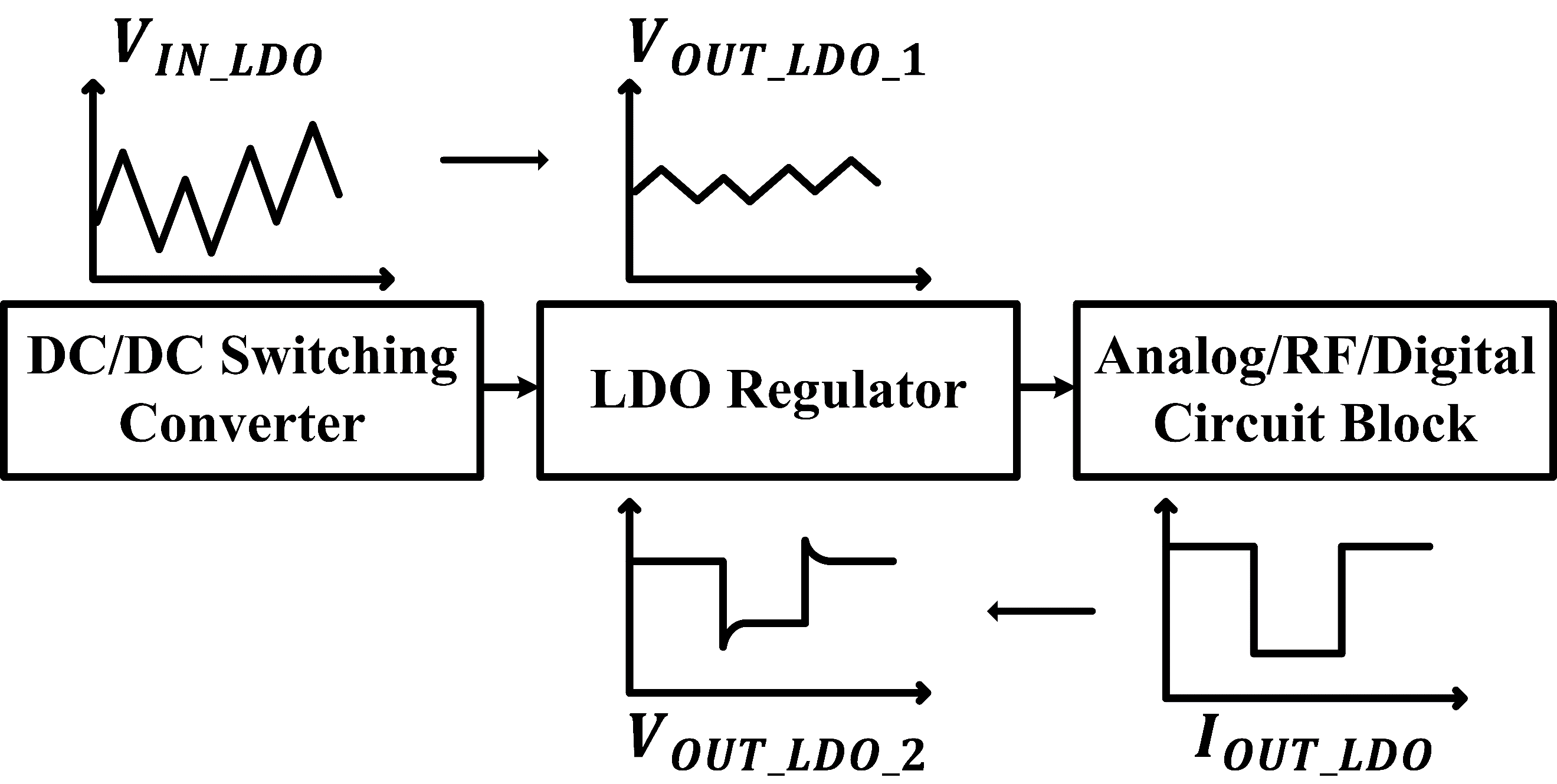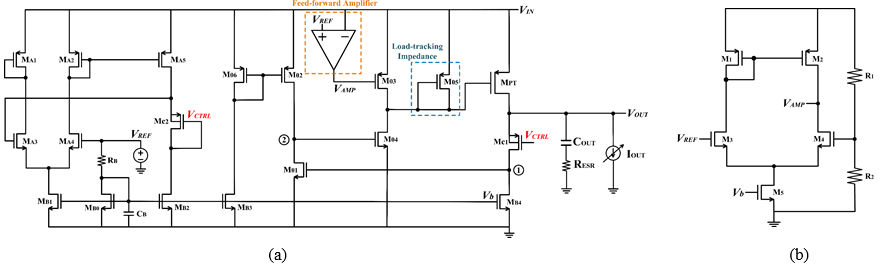Mobile QR CodeLow-dropout (LDO) regulator, power-supply rejection ratio (PSRR), fast transient response time, low output peak voltage

## I. INTRODUCTION

Analog applications and the device that deal with small signals continue to become smaller and more functional as the modern technology advance. Therefore, a LDO should provide the system with clean and ripple-free power because a voltage ripple can cause a fatal defect in the noise-sensitive RF/analog blocks. There are two reasons why the LDO supply voltage change: i) line regulation by input voltage swing; ii) load transient response by load current rapid change.

Fig. 1. Conceptual block diagram of typical power management system.Fig. 1 explains the line regulation and the load transient response. The line regulation is the ability to supply a less variable output voltage ($V_{OUT\_ LDO\_ 1}$) despite of changes in the input voltage ($V_{IN\_ LDO}$). The LDO regulator with a lower PSRR (i.e., $20\log \left(V_{OUT\_ LDO\_ 1}/V_{IN\_ LDO}\right)$) is efficient (1). The load transient response refers to how much the output voltage ($V_{OUT\_ LDO\_ 2})~$fluctuates when the step of load current ($I_{OUT\_ LDO}$) is present. The output transient response should not deviate significantly from the regulated value and should be promptly restored to a target voltage. As the output capacitor ($C_{OUT}$) increases, the transient response time also increases (2). When $C_{OUT}$ is reduced to achieve fast transient time, the equivalent-series-resistance ($R_{ESR}$) had to be large because stability must be ensured using pole-zero cancellation (i.e., $Z_{ESR}$=1/$C_{OUT}R_{ESR}$) (3). A high $R_{ESR}$ degrades the performance of a load transient response while inducing a high voltage spike (2).

In (4), fast transient response is obtained by adaptively biased error amplifier, however, the voltage spike is large. In (5), the overshoot is reduced, but the PSR is still low. The circuit in (6) achieves a -56 dB PSRR, but the load transient performance is not good because $R_{ESR}$ is as large as 30-m${\omega}$. Moreover, in previously introduced circuits in (7-9) with fast load transients, they show poor PSRR performance.

The output ripple by input ripple is suppressed using FFRC technique (6). In this paper, the structure is simpler than (6) by eliminating the summing amplifier that merges the feedback regulating loop with feed-forward path at the gate of the pass transistor. And, the non-dominant pole is moved behind the unity-gain frequency (UGF) by adding a load-tracking impedance at the gate of pass-transistor. Therefore, a stability is guaranteed while reducing both the transient response time and the output spike. The proposed LDO supplies a low-ripple and stable signal with little change even if the input voltage and output current are changed. Section Ⅱ discusses the structure and the implementation of circuit. The simulation results and performance comparison are shown in Section Ⅲ. Finally, the conclusions are provided in section Ⅳ.

## II. PROPOSED LDO ARCHITECTURE AND CIRCUIT IMPLEMENTATION

Fig. 2. (a) Schematic of proposed LDO structure, (b) Feed-forward amplifier.Fig. 2 presents the proposed LDO regulator. The proposed LDO applies a load-tracking impedance adjustment and loop-gain boosting technique with a feed-forward amplifier (6,7). In Fig. 2, $M_{PT}$ is the pass transistor and $M_{C1}$, $M_{B4}$, $M_{01}$ and $M_{02}$ constitute the folded common-gate amplifier. Similar to the design reported in (8), $M_{B1}$, $M_{B2}$ and $M_{A1}$-$M_{A5}$ constitute a control voltage (i.e., $V_{CTRL}$) generator for the gate voltage of $M_{C1}$. The reference voltage $V_{REF}$ (e.g., bandgap voltage reference), $R_{B}$ and $M_{B0}$ provide the bias current, and $C_{B}$ is a filtering capacitor.

$C_{OUT}$ and $R_{ESR}$ are reduced to improve load transient performance. Since this architecture has a 100-nF output capacitor which has low $R_{ESR}$, the Z_ESR is located after the UGF which is about 10-MHz. Therefore, $p_{2}$ generated by the gate of M_PT must also be located beyond the UGF, and only $p_{1}$ (i.e., {$C_{OUT}$ [($r_{o_PT}$ || ${g_{m,C1}}^{-1}$)]}$^{-1}$) must locate before the UGF. By reducing the resistance of the gate using diode-connected M_05, $p_{2}$ is also pushed back to 10-MHz. Even if the output current ($I_{OUT}$) and UGF increase, $g_{m,05}$ and $p_{2}$ also increase and the stability is ensured. M_03 and M_04 boost the gain that fell due to the load-tracking impedance. The poles at ①, ② must also be located beyond the UGF. The pole at ① is located at 107 MHz because $C_{g,01}$=1.7 fF, $r_{o,C1}$=1.64 MΩ and $r_{o,B4}$=1.84 MΩ. The pole at ② is located at 369 MHz because $C_{g,04}$=362 aF, $r_{o,01}$=3.2 MΩ, and $r_{o,02}$= 1.89 MΩ. Fig. 4 depicts the simulated loop gain-phase plot under different $I_{OUT}$ ranging from 2 mA to 10 mA. The phase margin is higher than 60˚.

With a mathematical model of the LDO shown in Fig. 3, the transfer gain from input to output is obtained as below.

##### (1)
$\frac{V_{OUT}}{V_{IN}}\left(s\right)=\frac{1+g_{m,MPT}r_{ds,MPT}\left\{1+PSRR_{e}+g_{m,03}\cdot R_{gate}\cdot H_{ff}\left(s\right)\right\}}{1+\frac{r_{ds,MPT}}{Z_{L}\left(s\right)}+g_{m,MPT}r_{ds,MPT}\cdot A_{C}\cdot A_{e}\cdot g_{m,04}\cdot R_{gate}}$ {\ldots}{\ldots}

where $PSRR_{e}$, $A_{C}$, $A_{e}$ and $R_{gate}$ are the power-supply rejection ratio of the error amplifier, $g_{m,C1}(r_{o,C1}||r_{o,B4}),$$g_{m,01}(r_{o,01}||r_{o,02})$and$(r_{o,03}||r_{o,04}||{g_{m,05}}^{- 1})$, respectively.

From Eq. (1), an optimal $H_{ff}\left(s\right)$ is figured out to prevent the output ripple for the input ripple. That is, the LDO transfer function must be zero for the enhanced PSRR (6).

##### (2)
$H_{ff}\left(s\right)|_{opt}=\frac{- \left(PSRR_{e}+\frac{1}{g_{m,MPT}r_{ds,MPT}}+1\right)}{g_{m,03}\cdot \left(r_{o,03}||r_{o,04}||{g_{m,05}}^{- 1}\right)}$

The PSRR greatly improves when the gain of the feed-forward amplifier is as shown in Eq. (2). The improvements will be introduced in the next section.

## III. SIMULATION RESULTS

Fig. 5 presents the simulated load transient responses of the proposed LDO regulator. The output voltage when $I_{OUT}$ is switched from 2 mA to 10 mA with 10 ns rise/fall times (∆t) is simulated. As a result, the undershoot/overshoot are less than 861$\mu \mathrm{V}$ and the response time is less than 35 ns.

Fig. 6 shows a comparison of the PSRR simulation results based on the presence or absence of FFRC. The PSRR is worse than 42-dB without feed-forward amplifier. By adding a feed-forward amplifier and removing the output ripple, 91-dB PSRR in DC and 70-dB PSRR at 46 MHz are obtained. After 46 MHz, the PSRR is improved again by the effect of $C_{OUT}$(1).

Table 1. Performance summary

 * *   *  This Work Tech. (nm) 130 350 180 350 65 40 65 $V_{IN}$ (V) > 1.15 1.5-3 1.35 5 0.7-1.2 1.1-1.9 1.2 $V_{OUT}$ (V) 1 1.2 1.2 1.8 0.66-1.16 0.2-1.1 1 $V_{do}$ (V) > 0.15 0.3-1.8 0.15 3.2 0.04-0.24 0.2 0.2 $I_{Q}$ (μ A) 50 26 15 N/A 116-874 56 27 $C_{OUT}$ (μ F) 4 0.1 0.22 N/A Cap-free 1 0.1 $R_{ESR}$ (m\omega) 30 23 1000 N/A N/A N/A ≈0 $T_r$ (μ s) N/A 0.2 N/A 4.92 0.077 N/A 0.035 PSRR (dB) @ Freq (Hz) -60@100k -67@1M N/A > -18 > -67.26 -18@100k -10@1M -60@1M -91.76@100k -91.33@1M $\frac{\Delta \mathrm{V}_{\mathrm{OUT}}}{\mathrm{V}_{\mathrm{OUT}}}$ rise/fall times 26.2 m 10 ns 56 m 100 ns 5.2 m N/A 5.96 m N/A 80 m-137 m N/A 140 m 900 ns 0.87 m 10 ns

*Measured value

The performance summary and comparison is shown in Table 1. The proposed LDO is sufficiently stabilized with a 100-nF output capacitor while (6,13) use a capacitor over 1$\mu \mathrm{F}$. $I_{Q}$ is not large when compared with others. The response time is 35 ns, which is the fastest compared to other structures. Finally, the proposed LDO regulator has an improved PSRR of -91~dB.

## IV. CONCLUSIONS

The proposed LDO regulator includes a feed-forward amplifier and a load tracking impedance. By applying the proposed idea, an improved PSRR and a low output peak voltage are achieved with 100-nF output capacitor which has low-ESR. Finally, this design provides a stable voltage quickly even if the input voltage and output current change.

### ACKNOWLEDGMENTS

This work was supported by Incheon National University Research Grant in 2018.

### REFERENCES

1
Teel John C., 2Q. 2005, Understanding power supply ripple rejection in linear regulators, Texas Instrum. Anal. Appl. J., pp. 8-102
King B. M., NOV 2000, Understanding the load-transient response of LDOs, Texas Instrum. Anal. Appl. J., pp. 19-233
Falin J., May. 2002, ESR, stability and the LDO regulator, Texas Instruments, Dallas, TX, Texas Instruments Application Report SLVA1154
Mandal D., Desai C., Bakkaloglu B., Kiaei S., Feb 2019, Adaptively Biased Output Cap-less NMOS LDO with 19 ns Settling Time, IEEE Transactions on Circuits and Systems II: Express Briefs, Vol. 66, pp. 167-1715
Chong S., Chan P. K., April 2013, A 0.9-μA Quiescent Current Output-Capacitorless LDO Regulator with Adaptive Power Transistors in 65-nm CMOS, IEEE Transactions on Circuits and Systems I: Regular Papers, Vol. 60, pp. 1072-10816
El-Nozahi M., Amer A., Torres J., Entesari K., Sanchez-Sinencio E., Mar 2010, High PSR low drop-out regulator with feedforward ripple cancellation technique, IEEE J. Solid-State Circuits, Vol. 45, No. 3, pp. 565-5777
Hung W.-J., Lu S.-H., Liu S.-I., Feb 2006, CMOS low dropout linear regulator with single Miller capacitor, Electron. Lett., Vol. 42, No. 4, pp. 216-2178
Or P. Y., Leung K. N., Oct 2010, A Fast-Transient Low-Dropout Regulator with Load-Tracking Impedance Adjustment and Loop-Gain Boosting Technique, IEEE Transactions on Circuits and Systems II: Express Briefs, Vol. 57, No. 10, pp. 757-7619
Tong X., Wei K., 2017, A fully integrated fast-response LDO voltage regulator with adaptive transient current distribution, IEEE Computer Society Annual Symposium on VLSI, Bochum: North rhine-Westphalia, pp. 651-65410
Cheng-Han H., Chung-Yen D., Shuenn-Yuh L., Jun 2014, Power management with energy harvesting from a headphone jack, IEEE International Symp. on Circuits and Systems (ISCAS), pp. 1989-1992, 1-511
Luo P., Liu Z., Huang L., Zhen S., Aug 2018, A Fast-Response NMOS-LDO Voltage Regulator without on-chip Compensated Capacitor, IEEE International Midwest Symposium on Circuits and Systems (MWSCAS), pp. 611-614, 5-812
Akram M. A., Hong W., Hwang I.-C., Jan 2019, Capacitorless Self-Clocked All-Digital Low-Dropout Regulator, IEEE Journal of Solid-State Circuits, Vol. 54, No. 1, pp. 266-27613
Huang C.-H., Liao W.-C., May 2020, A high-performance LDO regulator enabling low-power SoC with voltage scaling approaches, IEEE Trans. Very Large Scale Integr. (VLSI) Syst., Vol. 28, No. 5, pp. 1141-1149## Author

Nahyun Kim was born in Incheon, Korea, in 1998.

She will receive the B.S degree in electronic engineering from the Incheon National University, Korea, in 2022.

Ms. Kim received the Excellence Award at the result presentation of the “Advanced Course for Women’s Graduate School Student Engineering Research Team” under the theme of "Low-Power Decision Feedback Equalizer for 28Gb/s Interface in 65nm CMOS Process" in 2020.

Junyoung Song (Member, IEEE) received the B.S. and M.S. degrees in electronics engineering and the Ph.D. degree in electrical and computer engineering from Korea University, Seoul, South Korea, in 2008, 2010, and 2014, respectively.

In 2012, he was a Visiting Scholar with the University of California at Los Angeles, Los Angeles, CA, USA.

In 2014, he joined the Analog Serial I/O Group, Intel Corporation, San Jose, CA, where he was involved in the wireline transceiver design for high-performance FPGA.

Since 2018, he has been with the School of Electronics Engineering, Incheon National University, Incheon, South Korea, where he is currently an Assistant Professor.

He has coauthored the book High-Bandwidth Memory Interface (Springer, 2013).

His research interests include the high-speed wireline transceiver, memory, and clock generator.

Dr. Song was a recipient of the Minister of Ministry of Education, Science and Technology Award at the Korea Semiconductor Design Contest in 2011 and the IEEE Seoul Section Student Paper Contest Bronze Award in 2011 and 2013.

He is serving on the Technical Program Committee of the IEEE Asian Solid-State Circuits Conference.